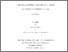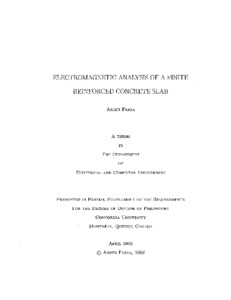Title:

# Electromagnetic analysis of a finite reinforced concrete slab

Parsa, Armin (2008) Electromagnetic analysis of a finite reinforced concrete slab. PhD thesis, Concordia University.Preview
Text (application/pdf)
NR37758.pdf - Accepted Version
2MB

## Abstract

In order to calculate the electromagnetic fields reflected, transmitted, and diffracted by a finite reinforced concrete slab in the presence of a source, a Green's function/method of moments approach has been developed. In doing so, a Green's function solution for a finite and electrically thick dielectric slab is obtained. The Green's function for the arbitrary position of the source and field point is based on the interior Green's function, i.e. the Green's function when the source and the field point are both inside the slab. The solution is two-dimensional, with an electric line source excitation. The first development in this thesis presents an interior electric field Green's function for a thick and finite dielectric slab. The presented solution is based on the separation of variables method which gives an exact solution to a separable slab. The separable slab is closely related to the finite slab. The separable slab solution is expressed in terms of the contribution of the surface wave modes plus the remaining part which is called the "residual wave"' contribution. In order to model a finite slab, the surface wave contribution of the separable slab is modified since the separable slab solution fails to model the finite slab when a surface wave mode is close to resonance. The modification is done by correcting the end cap reflection coefficient for each mode and accounting for the mode conversions. The mode conversions and reflections are characterized by the end cap scattering matrix which is obtained by the method of moments. As a result, the interior Green's function solution for a finite slab is the modified surface wave solution plus the residual wave contribution obtained for the separable slab. The Green's functions for the cases when the source and/or field points are outside the slab are obtained using the interior Green's function and the surface equivalence principle. Having the finite slab Green's function, it is possible to model a finite reinforced concrete slab. Since the metallic rods are assumed to be electrically thick, each metallic rod is replaced by a circular array of thin wires complying with the "same surface area" rule of thumb. To obtain the unknown induced currents on the surface of the thin wires, the method of moments is used in conjunction with the finite slab Green's function. The model is used to investigate the reflection and transmission of electromagnetic waves for some cases of practical interest

Divisions: Concordia University > Gina Cody School of Engineering and Computer Science > Electrical and Computer Engineering Thesis (PhD) Parsa, Armin xx, 163 leaves : ill. ; 29 cm. Concordia University Ph. D. Electrical and Computer Engineering 2008 Paknys, Robert 975882 Concordia University Library 22 Jan 2013 16:16 18 Jan 2018 17:41 http://clues.concordia.ca/search/c?SEARC...
All items in Spectrum are protected by copyright, with all rights reserved. The use of items is governed by Spectrum's terms of access.

Repository Staff Only: item control pageResearch related to the current document (at the CORE website)
Back to top